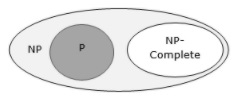# What is NP-completeness in TOC?

The Non-deterministic Polynomial (NP) problems were a little harder to understand. In terms of solving a NP problem, the run-time is not polynomial. It would be something like O(n!) or something larger.

However, this class of problems are given a specific solution, and checking the solution would have a polynomial run-time.

For example, the Sudoku game.

## NP-Hard Problems

A problem is said to be NP-Hard when an algorithm for solving NP Hard can be translated to solve any NP problem. Then we can say, this problem is at least as hard as any NP problem, but it could be much harder or more complex.

## NP-Complete Problems

NP-Complete (NPC) problems are problems that are present in both the NP and NP-Hard classes. That is NP-Complete problems can be verified in polynomial time and any NP problem can be reduced to this problem in polynomial time.

A problem is in class NPC if it is in NP and is as hard as any problem in NP. A problem is said to be NP-hard if all problems in NP are polynomial time reducible to it, even though it may not be in NP itself.

If a polynomial time algorithm exists for any of these types of problems, all problems in NP can be polynomial time solvable. These problems are called NP-complete. NPcompleteness is important for both theoretical and practical reasons.

## Definition of NP-Completeness

A language M is NP-complete, if it satisfies the two conditions which are given below −

• M is in NP.

• Every A in NP is polynomial time reducible to M.Suppose, if a language satisfies the second property, but not necessarily the first one, the language M is known as NP-Hard.

Informally, a search problem M is NP-Hard if there exists some NP-Complete problem A that Turing reduces to M.

## NP-Complete Problems

Examples of NP-Complete problems where no polynomial time algorithm is known are as follows −

• Determining whether a graph has a Hamiltonian cycle

• Determining whether a Boolean formula is satisfactory, etc.

## NP-Hard Problems

The following problems are NP-Hard

• The circuit-satisfiability problem

• Set Cover

• Vertex Cover

• Travelling Salesman Problem